# Implementing a Regular Expression Engine

Understanding and using regular expressions properly is a valuable skill when it comes to text processing. Due to their declarative yet idiomatic syntax, regular expressions can sometimes be a source of confusion (even anxiety) amongst software developers. In this article, we’ll implement a simple and efficient regex engine. We’ll define the syntax of our regular expressions, learn how to parse them and build our recognizer. First, we’ll briefly cover some theoretical foundations.

## Finite Automata

In informal terms finite automaton (or finite state machine) is an abstract machine that has states and transitions between these states. It is always in one of its states and while it reads an input it switches from state to state. It has a start state and can have one or more end (accepting) states.

### Deterministic Finite Automata (DFA)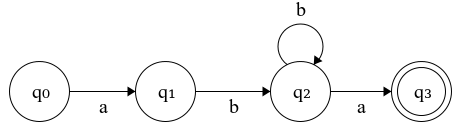Figure 1.1: Deterministic Finite Automaton (DFA)

In Fig 1.1 we have automaton with four states; $$q_0$$ is called the start state and $$q_3$$ is the end (accepting) state. It recognizes all the strings that start with “ab”, followed by an arbitrary number of ‘b’ s and ending with an ‘a’.

If we process the string “abba” through the machine on Fig 1.1 we’ll go through the following states:

Step  State   Input   New State
0 $$q_0$$ a $$q_1$$
1 $$q_1$$ b $$q_2$$
2 $$q_2$$ b $$q_2$$
3 $$q_2$$ a $$q_3$$

For the strings “aba”, “abbba” or “abbbbba”, the automaton will end up in the accepting state of $$q_3$$. If at any point during processing, the machine has no state to follow for a given input symbol - it stops the execution and the string is not recognized. So it won’t recognize “ab” as it will end up in the non-accepting state of $$q_2$$ and “abca” as there’s no transition on the symbol ‘c’ from $$q_2$$. In the example of Fig 1.1, at each state for a given valid input symbol, we can end up in exactly one state, we say that the machine is deterministic (DFA).

### Nondeterministic Finite Automata (NFA)

Suppose we have the following automaton: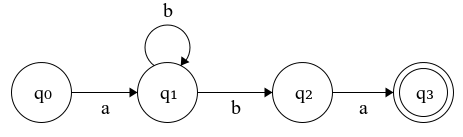Figure 1.2: Nondeterministic Finite Automaton (NFA)

We can see on Fig 1.2 that from $$q_1$$ on input ‘b’ we can transition to two states - $$q_1$$ and $$q_2$$. In this case, we say that the machine is nondeterministic (NFA). It is easy to see that this machine is equivalent to the one in Fig 1.1, i.e. they recognize the same set of strings. Every NFA can be converted to its corresponding DFA, the proof and the conversion, however, are a subject of another article.

### ε-NFA

We represent an ε-NFA exactly as we do an NFA but with one exception. It includes transitions on the empty string - ε. That means from one state we are able to transition into another without reading an input symbol. These transitions are usually denoted with the Greek letter ε (epsilon).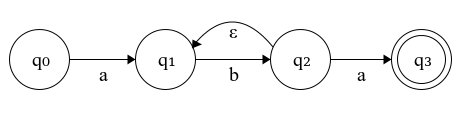Figure 1.3: epsilon-NFA

On Fig 1.3 we can see that we have ε-transition from $$q_2$$ to $$q_1$$. This ε-NFA is equivalent to the NFA in Fig 1.2.

## Compiling Regular Expressions to Finite Automata

The set of strings recognized by a finite automaton $$A$$ is called the language of $$A$$ and is denoted as $$L(A)$$. If a language can be recognized by a finite automaton then there’s is a corresponding regular expression that describes the same language and vice versa (Kleene’s Theorem). The regular expression, equivalent to the automaton in Fig 1.1 would be abb*a. In other words, regular expressions can be thought of as a user-friendly alternative to finite automata for describing patterns in text.

### Thompson’s Construction

Algebras of all kinds start with some elementary expressions, usually constants and/or variables. Then they allow us to construct more complex expressions by applying a certain set of operations to these elementary expressions. Usually, some method of grouping operators with their operands such as parentheses is required as well.
For instance, in the arithmetic algebra we start with constants such as integers and real numbers, we include variables and using arithmetic operators, such as +, ×, we build more complex expressions. The regular expressions are in a way no different. Using constants, variables, and operators as building blocks, they denote formal languages (sets of strings).

We’ll describe an implementation by Ken Thompson presented in his paper Regular Expression Search Algorithm (1968).

To compile a regular expression $$R$$ to an NFA we first need to parse $$R$$ into its constituent subexpressions. The rules for constructing an NFA can be split into two parts:

1. Base rules for handling subexpressions with no operators.
2. Inductive rules for constructing larger NFAs from the smaller NFAs by applying the operators.

#### Basis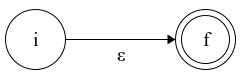Figure 2.1: Finite automaton for the expression ε

On Fig 2.1 we have an automaton that recognizes the empty string ε. $$i$$ is the start state and $$f$$ is the accepting state.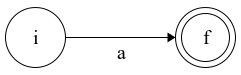Figure 2.2: Finite automaton for the expression a

On Fig 2.2 we construct an automaton for the symbol ‘a’. We treat each symbol of the input alphabet as a regular expression by itself. The language of this automaton consists only of the word “a”.

#### Induction

Suppose we have the two regular expressions $$S$$ and $$T$$ and their NFAs $$N(S)$$ and $$N(T)$$ respectively:

a) Union: $$R = S \vert T$$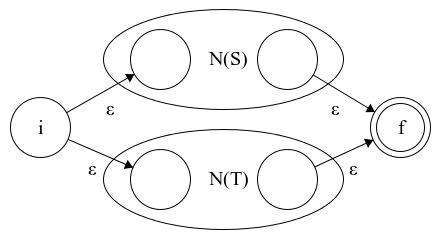Figure 3.1: Union of two NFAs

We introduce a start state $$i$$ and add ε-trainsitions from it to the start states of $$N(S)$$ and $$N(T)$$. Next, we add transitions from the end states of $$N(S)$$ and $$N(T)$$ to the newly created $$f$$ state and mark them as not accepting. The resulting NFA will recognize strings that are either belong to $$L(S)$$ or $$L(T)$$.

b) Concatenation: $$R = S \cdot T$$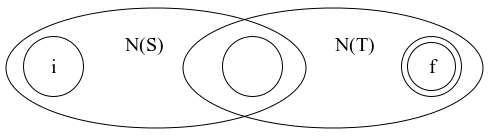Figure 3.2: Concatenation of two NFAs

We mark the accepting state of $$N(S)$$ as not accepting and add a transition from it to the start state of $$N(T)$$. Here $$i$$ denotes the start state of $$N(S)$$ and $$f$$ denotes the accepting state of $$N(T)$$. This would result in an NFA that recognizes all the string concatenations $$vw$$ where $$v$$ belongs to $$L(S)$$ and $$w$$ belongs to $$L(T)$$.

c) Closure (Kleene Star): $$R = S \ast$$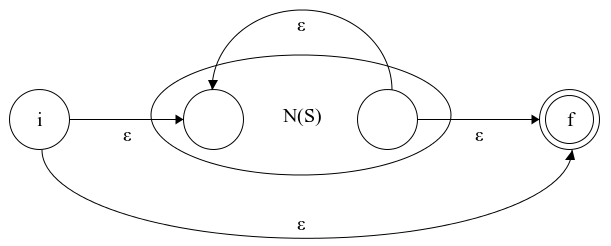Figure 3.3: NFA for the closure of a regular expression.

We introduce $$i$$ as start and $$f$$ as an accepting state. We add ε-transitions: from $$i$$ to $$f$$, from $$i$$ to the start state of $$N(S)$$, then we connect the accepting state of $$N(S)$$ with $$f$$ and finally add a transition from the end state of $$N(S)$$ to its start state. We mark the end state of $$N(S)$$ as intermediate.

The closure (*) operator has the highest precedence, followed by concatenation $$(\cdot)$$. The union $$(\vert)$$ is the operation with the lowest precedence. Modern regex implementations have additional operators like + (one or more), ? (zero or one), their implementation, however, is analogous to the ones above and we’ll skip them for the sake of brevity.

#### Example

Let’s go through an example case. We want to construct an NFA for (a∣b)*c. The language of this expression contains all the strings that have zero or more ‘a’ s or ‘b’ s and end with ‘c’. Just like in arithmetic expressions, we use brackets to explicitly specify the operator precedence. We break the expression into its atomic subexpressions and build our way up. By the order of precedence we:

1. Construct $$N(a)$$: NFA for ‘a’.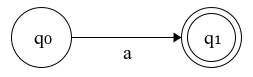Figure 4.1: N(a): NFA for 'a'

1. Construct $$N(b)$$: a NFA for ‘b’.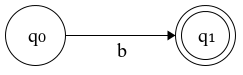Figure 4.2: N(b): NFA for 'b'

1. Apply union on $$N(a)$$ and $$N(b)$$ → $$N(a \vert b)$$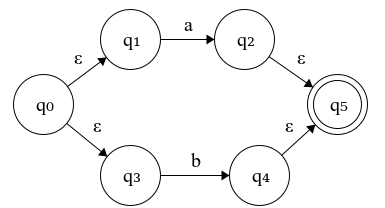Figure 4.3: N(a∣b): Union of N(a) and N(b)

1. Apply closure on $$N(a|b)$$ → $$N((a \vert b)\ast)$$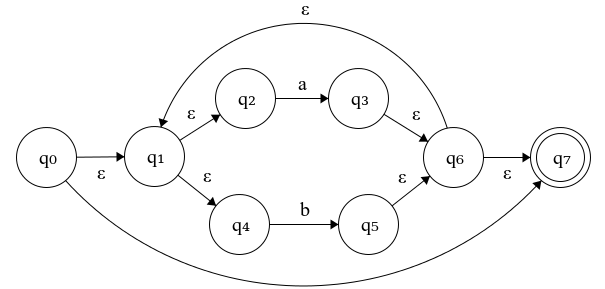Figure 4.4: N((a∣b)*): Closure of N(a∣b)

1. Apply concatenation to $$N((a \vert b)*)$$ with $$N(c)$$. The construction of $$N(c)$$ is analogous to steps 1) and 2).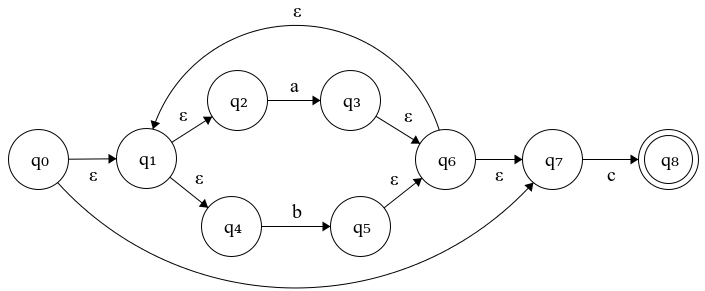Figure 4.5: N((a∣b)*c): NFA for the expression of (a∣b)*c

### Parsing a regular expression

First, we need to preprocess the string by adding an explicit concatenation operator. We’re going to use the dot (.) symbol, as described in the paper. So for example, the regular expression $$abc$$ would be converted to $$a \cdot b \cdot c$$ and $$(a \vert b)c$$ wound turn into $$(a \vert b)\cdot c$$ You can check the implementation here.

The modern implementations use the dot character as “any” metacharacter. They would also probably build the NFA during parsing instead of creating a postfix expression still doing it this way would let us understand the process more clearly.

There are several ways of parsing a regular expression. We’ll follow through Thompson’s original paper and that is by converting our expression from infix into postfix notation. This way we can easily apply the operators in the defined order of precedence.

We won’t delve into the technical details of this algorithm. You can check my implementation in less than 40 lines of javascript here and a neat explanation with more examples here.

### Constructing the NFA

We represent an NFA state as an object with the following properties:

function createState(isEnd) {
return {
isEnd,
transition: {},
epsilonTransitions: []
};
}


There are two types of transitions - by a symbol and by epsilon (empty string). A state in Thompson’s NFA can either have a symbol transition to at most one state or epsilon transitions to up to two states, but it cannot have a symbol and epsilon transitions at the same time.

function addEpsilonTransition(from, to) {
from.epsilonTransitions.push(to);
}

from.transition[symbol] = to;
}


From our basis, we have two types of NFA’s that would serve as our building blocks: an ε-NFA and a symbol-NFA. This is how we implement them:

function fromEpsilon() {
const start = createState(false);
const end = createState(true);

return { start, end };
}

function fromSymbol(symbol) {
const start = createState(false);
const end = createState(true);

return { start, end };
}


The NFA is simply an object which holds references to its start and end states. By following the inductive rules (described above), we build larger NFAs by applying the three operations on smaller NFAs.

function concat(first, second) {
first.end.isEnd = false;

return { start: first.start, end: second.end };
}

function union(first, second) {
const start = createState(false);

const end = createState(true);
first.end.isEnd = false;
second.end.isEnd = false;

return { start, end };
}

function closure(nfa) {
const start = createState(false);
const end = createState(true);

nfa.end.isEnd = false;

return { start, end };
}


Now is time to put it all together. We scan our postfix expression one symbol at a time and store the context in a stack. The stack contains NFAs.

• When we scan a character - we construct a character-NFA and push it to the stack.
• When we scan an operator, we pop from the stack, apply this operation on the NFA(s) and push the resulting NFA back to the stack.
function toNFA(postfixExp) {
if(postfixExp === '') {
return fromEpsilon();
}

const stack = [];

for (const token of postfixExp) {
if(token === '*') {
stack.push(closure(stack.pop()));
} else if (token === '|') {
const right = stack.pop();
const left = stack.pop();
stack.push(union(left, right));
} else if (token === '.') {
const right = stack.pop();
const left = stack.pop();
stack.push(concat(left, right));
} else {
stack.push(fromSymbol(token));
}
}

return stack.pop();
}


#### Example

Let’s simulate the algorithm on (a∣b)*c

1. Insert explicit concatenation operator (.): (a∣b)*c(a∣b)*.c
2. Convert to postfix notation: (a∣b)*.cab∣*c.
3. Construct an NFA:
Step     Scan      Operations  Stack
0 ab∣*c. fromSymbol(a); push; { N(a) }
1 ab∣*c. fromSymbol(b); push; { N(a), N(b) }
2 ab*c. pop; pop; union(N(a), N(b)); push;     { N(a∣b) }
4 ab∣*c. pop; closure(N(a∣b)); push; { N((a∣b)*) }
5 ab∣*c. fromSymbol(c); push; { N((a∣b)*), N(c) }
6 ab∣*c. pop; pop; concat(N((a∣b)*), N(c)); push;    { N((a∣b)*c) }

## NFA Search Algorithms

### Recursive Backtracking

The simplest way to check if a string is recognized by automaton is by computing all the possible paths through the NFA until we end up in an accepting state or exhaust all the possibilities. It falls down to a recursive depth-first search with backtracking. Let’s see an example: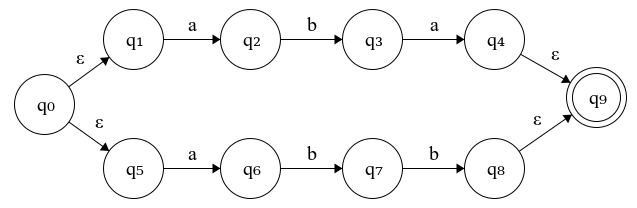Figure 5.1: NFA for the expression (aba)∣(abb)

The automaton in Fig. 5.1 recognizes either the string “aba” or “abb”. If we want to process “abb”, our simulation with recursive backtracking would process the input one state at a time so we’ll first end up reaching $$q_3$$ after reading “ab” from the input string. The next symbol is ‘b’ but there’s no transition from $$q_3$$ on ‘b’, therefore, we backtrack to $$q_0$$ and take the other path which leads us to the accepting state. You can check out my implementation on GitHub.

For the automaton on Fig 5.1 we ended up going through all of the possible two paths. This doesn’t seem like a big deal but in a more complex scenario, there might be considerable performance implications.

Given an NFA with n states, from each of its states, it can transition to at most n possible states. This means there might be a maximum $$2^n$$ paths, thus in the worst case this algorithm will end up going through all of these paths until it finds a match (or not). Needless to say, O($$2^n$$) is not scalable because for a string of 10 characters to check whether it’s matched or not we might end up doing 1024 operations. We should certainly do better than that.

### Being in multiple states at once

We can represent an NFA to be at multiple states at once as described in Thompson’s paper. This approach is more complex but produces significantly better performance.

When reading a symbol from the input string, instead of transitioning one state at a time we’ll transition into all the possible states, reachable from the current set of states. In other words, the current state of the NFA will actually become a set of states. Each turn after reading a symbol, for each state in the current set, we find the states that can be transitioned into using that symbol and mark them as the next states. So with this approach, our simulation for “abb” would be:

Step Current Scan Next
1 { $$q_1$$, $$q_5$$ } abb { $$q_2$$, $$q_6$$ }
2 { $$q_2$$, $$q_6$$ } abb { $$q_3$$, $$q_7$$ }
3 { $$q_3$$, $$q_7$$ } abb { $$q_9$$ }

We check if any state in the set of states that we end up with is an accepting state and if so - the string is recognized by the NFA. In the above case, the final set contains only $$q_9$$ which is an accepting state.

#### Dealing with ε-transitions

As mentioned above, Thompson’s construction has two types of states. Ones with ε-transitions and ones with a transition on a symbol. So each time we end up in a state with ε-transition(s) we simply follow through to the next state(s) until we end up in one that has a transition on a symbol and insert it to the set of next states. This is obvious on step 3 from the example above, on $$q_7$$ we read ‘b’ and proceed to $$q_8$$ which is an epsilon transition state. We follow the ε-transition and reach $$q_9$$ which has no ε-transitions so we add it to the list of next states. This is a recursive procedure.

function addNextState(state, nextStates, visited) {
if (state.epsilonTransitions.length) {
for (const st of state.epsilonTransitions) {
if (!visited.find(vs => vs === st)) {
visited.push(st);
}
}
} else {
nextStates.push(state);
}
}


We also have to mark the ε-transition states as visited to prevent infinite looping. The bottom of the recursion is when we reach a state with no ε-transitions. This is the code or the search procedure:

function search(nfa, word) {
let currentStates = [];

for (const symbol of word) {
const nextStates = [];

for (const state of currentStates) {
const nextState = state.transition[symbol];
if (nextState) {
}
}
currentStates = nextStates;
}

return currentStates.find(s => s.isEnd) ? true : false;
}


The initial set of current states is either the start state itself or the set of states reachable by epsilon transitions from the start state. In the example on Fig 5.1 the start state $$q_0$$ is an ε-transition state, so we follow the transitions recursively until reaching the symbol transition states $$q_1$$ and $$q_5$$ which become our initial set of states.

Given a string of length n, on each iteration, this algorithm keeps two lists of states with a length of approximately n. This gives us a time complexity of $$O(n^2)$$ which significantly outperforms the recursive backtracking approach. We can optimize it by converting the NFA to an epsilon-free NFA and even further by converting it to a DFA. DFAs guarantee $$O(n)$$ recognition complexity but we might end up having $$2^n$$ states due to the state explosion problem.

## Putting it all together

function createMatcher(exp) {
const postfixExp = toPostfix(insertExplicitConcatOperator(exp));
const nfa = toNFA(postfixExp);

return word => search(nfa, word);
}

const match = createMatcher('a*b');
match(''); // false
match('b'); // true
match('ab'); // true


## Recap

We started by learning about finite automata and how do they work. We classified them as deterministic and nondeterministic. We also introduced the concept of ε-NFAs which are a specific type of nondeterministic automata. We’ve seen how NFAs and DFAs have the same expressive power and how they can be used for string recognition.

We defined the building blocks of the regular expressions and learned how by applying operators (concatenation, union, and closure) we can construct more complex expressions from smaller ones. Then we learned how to compile a regular expression into its equivalent NFA then implemented an algorithm that processes a string through an NFA to determine whether it is matched or not.